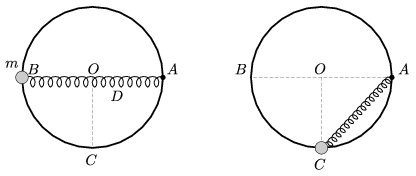Mathematical and Physical Journal
for High Schools
Issued by the MATFUND Foundation
 Already signed up? New to KöMaL?

#Problem P. 4405. (January 2012)

P. 4405. A small object of mass m=0.4 kg is threaded onto a vertical ring of radius r=30 cm, and of centre O. The object can move frictionless along the ring. One end of a spring of spring constant D=16 N/m, and of unstretched length 30 cm is attached to the small object and its other end is fixed to point A on the horizontal diameter of the path. Find the speed of the object when it passes the lowest point of the path C, if it is released from rest at point B. In this case what is the force exerted by the object on the ring, and what is the direction of its acceleration at point C?(4 pont)

Deadline expired on February 10, 2012.

Sorry, the solution is available only in Hungarian. Google translation

Megoldás. A test sebessége:

$\displaystyle v_{C}=\sqrt{2gr+2r^2\frac{D}{m}(\sqrt{2}-1)}=2{,}98~\frac{\rm m}{\rm s}.$

A gyűrűre ható erő a $\displaystyle C$ pontban:

$\displaystyle N=3mg+Dr\left( \frac{5}{\sqrt{2}}-3\right)=14{,}34~\rm N.$

A test gyorsulásvektora a pálya legalsó pontjában

$\displaystyle arcctg \left(\sqrt{8}+\frac{\sqrt{8}}{\sqrt{2}-1}\,\frac{mg}{Dr}\right)\approx 6{,}8^\circ$

szöget zár be a függőlegessel (és ,,felfelé" mutat).

### Statistics:

 139 students sent a solution. 4 points: Agócs Fruzsina, Barta Szilveszter Marcell, Bingler Arnold, Bognár Tamás, Bolgár Dániel, Büki Máté, Csáky Pál, Csathó Botond, Czipó Bence, Dávid Bence, Epinger Bálint, Fehér Zsombor, Ferenczi András, Filep Gábor, Garami Anna, Gróf Gábor, Horicsányi Attila, Horváth Dániel, Huszár Emese, Janzer Barnabás, Janzer Olivér, Juhász Péter, Kollarics Sándor, Laczkó Zoltán Balázs, Mihály 930 Ákos, Nagy Zsolt, Olosz Balázs, Papp Roland, Pázmán Zalán, Pölöskei Péter Zsolt, Seress Dániel, Simig Dániel, Szélig Áron, Szigeti Bertalan György, Tóth Bálint. 3 points: 34 students. 2 points: 33 students. 1 point: 11 students. 0 point: 18 students. Unfair, not evaluated: 8 solutionss.

Problems in Physics of KöMaL, January 2012